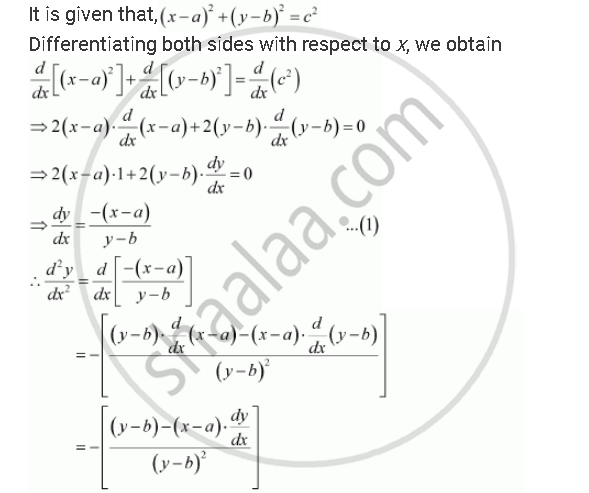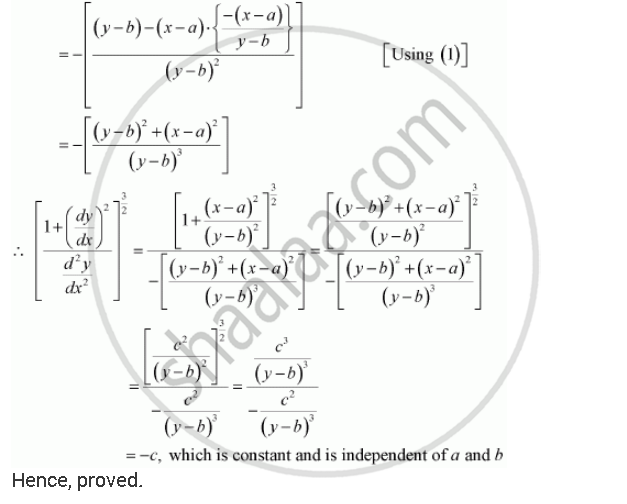Share

# If (X – A)2 + (Y – B)2 = C2, for Some C > 0, Prove that Is a Constant Independent Of A And B. - CBSE (Commerce) Class 12 - Mathematics

#### Question

If (x – a)2 + (y – b)2 = c2, for some c > 0, prove that

[1+ (dy/dx)^2]^(3/2)/((d^2y)/dx^2) is a constant independent of a and b.

#### SolutionIs there an error in this question or solution?

#### APPEARS IN

NCERT Solution for Mathematics Textbook for Class 12 (2018 to Current)
Chapter 5: Continuity and Differentiability
Q: 15 | Page no. 191

#### Video TutorialsVIEW ALL 

Solution If (X – A)2 + (Y – B)2 = C2, for Some C > 0, Prove that Is a Constant Independent Of A And B. Concept: Concept of Differentiability.
S